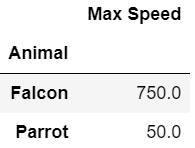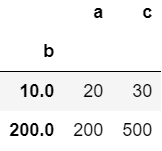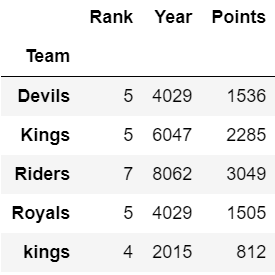# Pandas Groupby and Sum

• Last Updated : 25 Nov, 2020

Pandas is an open-source library that is built on top of NumPy library. It is a Python package that offers various data structures and operations for manipulating numerical data and time series. It is mainly popular for importing and analyzing data much easier. Pandas is fast and it has high-performance & productivity for users.

Groupby is a pretty simple concept. We can create a grouping of categories and apply a function to the categories. It’s a simple concept but it’s an extremely valuable technique that’s widely used in data science. It is helpful in the sense that we can :

• Compute summary statistics for every group
• Perform group-specific transformations
• Do the filtration of data

The groupby() involves a combination of splitting the object, applying a function, and combining the results. This can be used to group large amounts of data and compute operations on these groups such as sum().

Example 1:

## Python3

 `# import required module``import` `pandas as pd`` ` `# create dataframe``df ``=` `pd.DataFrame({``'Animal'``: [``'Falcon'``, ``'Falcon'``, ``'Parrot'``, ``'Parrot'``],``                   ``'Max Speed'``: [``380.``, ``370.``, ``24.``, ``26.``]})`` ` `# use groupby() to compute sum``df.groupby([``'Animal'``]).``sum``()`

OutputExample 2:

## Python3

 `# import required module``import` `pandas as pd`` ` `# assign list``l ``=` `[[``100``, ``200``, ``300``], [``10``, ``None``, ``40``],``     ``[``20``, ``10``, ``30``], [``100``, ``200``, ``200``]]`` ` `# create dataframe``df ``=` `pd.DataFrame(l, columns``=``[``"a"``, ``"b"``, ``"c"``])`` ` `# use groupby() to generate sum``df.groupby(by``=``[``"b"``]).``sum``()`

Output:Example 3:

## Python3

 `# import required module``import` `pandas as pd`` ` `# assign data``ipl_data ``=` `{``'Team'``: [``'Riders'``, ``'Riders'``, ``'Devils'``, ``'Devils'``,``                     ``'Kings'``,  ``'kings'``, ``'Kings'``, ``'Kings'``,``                     ``'Riders'``, ``'Royals'``, ``'Royals'``, ``'Riders'``],``            ``'Rank'``: [``1``, ``2``, ``2``, ``3``, ``3``, ``4``, ``1``, ``1``, ``2``, ``4``, ``1``, ``2``],`` ` `            ``'Year'``: [``2014``, ``2015``, ``2014``, ``2015``, ``2014``, ``2015``, ``2016``, ``                     ``2017``, ``2016``, ``2014``, ``2015``, ``2017``],`` ` `            ``'Points'``: [``876``, ``789``, ``863``, ``673``, ``741``, ``812``, ``756``, ``788``, ``                       ``694``, ``701``, ``804``, ``690``]}`` ` `# create dataframe``df ``=` `pd.DataFrame(ipl_data)`` ` `# use groupby() to generate sum``df.groupby([``'Team'``]).``sum``()`

Output:My Personal Notes arrow_drop_up